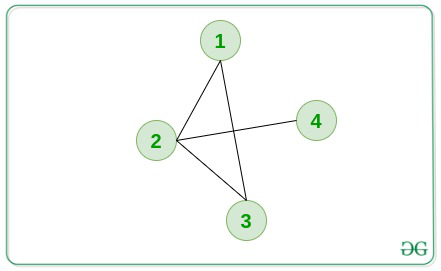# Minimum length paths between 1 to N including each node

• Last Updated : 15 Sep, 2021

Given an undirected graph consisting of N nodes and M edges, the task is to find the minimum length of the path from Node 1 to Node N passing from every possible node of the given graph. If there doesn’t exist any such path, then print -1.

Note: The path can pass through a node any number of times.

Examples:

Input: N = 4, M = 4, edges[][] = {{1, 2}, {2, 3}, {1, 3}, {2, 4}}
Output: 2 2 3 2
Explanation:Minimum path length from 1 to 4, passing from 1 is 2.
Minimum path length from 1 to 4, passing from 2 is 2.
Minimum path length from 1 to 4, passing from 3 is 3.
Minimum path length from 1 to 4, passing from 4 is 2.

Input: N = 5, M = 7, edges[][] = {{1, 2}, {1, 4}, {2, 3}, {2, 5}, {4, 3}, {4, 5}, {1, 5}}
Output: 1 2 4 2 1

Approach: The idea is to run two BFS, one from node 1 excluding node N and another from node N excluding node 1 to find the minimum distance of all the nodes from 1 and N. The sum of both the minimum distances will be the minimum length of the path from 1 to N including the node. Follow the steps below to solve the problem:

• Initialize a queue, say queue1 to perform BFS from node 1 and a queue queue2 to perform BFS from node N.
• Initialize two arrays, say dist1[] and dist2[] that store the shortest distance by performing BFS1 and BFS2.
• Perform two BFS and perform the following steps in each case:
• Pop from the queue and store node in x and its distance in dis.
• If dist[x] is smaller than dis then continue.
• Traverse the adjacency list of x and for each child y, if dist[y] is greater than dis + 1 then update dist[y] equals dis + 1.
• After populating the two arrays dist1[] and dist2[] in the above steps, iterate over the range [0, N] and if the sum of (dist1[i] + dist2[i]) is greater than 109 then print “-1” as their exists no such path. Otherwise, print the value of (dist1[i] + dist2[i]) as the result.

Below is the implementation of the above approach:

## C++

 `// C++ program for the above approach` `#include ``using` `namespace` `std;``#define ll long long int` `// Function to calculate the distances``// from node 1 to N``void` `minDisIncludingNode(``int` `n, ``int` `m,``                         ``int` `edges[])``{``    ``// Vector to store our edges``    ``vector g;` `    ``// Storing the edgees in the Vector``    ``for` `(``int` `i = 0; i < m; i++) {``        ``int` `a = edges[i] - 1;``        ``int` `b = edges[i] - 1;``        ``g[a].push_back(b);``        ``g[b].push_back(a);``    ``}` `    ``// Initialize queue``    ``queue > q;``    ``q.push({ 0, 0 });``    ``vector<``int``> dist(n, 1e9);``    ``dist = 0;` `    ``// BFS from first node using queue``    ``while` `(!q.empty()) {``        ``auto` `up = q.front();` `        ``// Pop from queue``        ``q.pop();``        ``int` `x = up.first;``        ``int` `lev = up.second;``        ``if` `(lev > dist[x])``            ``continue``;``        ``if` `(x == n - 1)``            ``continue``;` `        ``// Traversing its adjacency list``        ``for` `(ll y : g[x]) {``            ``if` `(dist[y] > lev + 1) {``                ``dist[y] = lev + 1;``                ``q.push({ y, lev + 1 });``            ``}``        ``}``    ``}``    ``// Initialize queue``    ``queue > q1;``    ``q1.push({ n - 1, 0 });``    ``vector<``int``> dist1(n, 1e9);``    ``dist1[n - 1] = 0;` `    ``// BFS from last node using queue``    ``while` `(!q1.empty()) {``        ``auto` `up = q1.front();` `        ``// Pop from queue``        ``q1.pop();``        ``int` `x = up.first;``        ``int` `lev = up.second;``        ``if` `(lev > dist1[x])``            ``continue``;``        ``if` `(x == 0)``            ``continue``;` `        ``// Traversing its adjacency list``        ``for` `(ll y : g[x]) {``            ``if` `(dist1[y] > lev + 1) {``                ``dist1[y] = lev + 1;``                ``q1.push({ y, lev + 1 });``            ``}``        ``}``    ``}` `    ``// Printing the minimum distance``    ``// including node i``    ``for` `(``int` `i = 0; i < n; i++) {``        ``// If not reachable``        ``if` `(dist[i] + dist1[i] > 1e9)``            ``cout << -1 << ``" "``;` `        ``// Path exists``        ``else``            ``cout << dist[i] + dist1[i] << ``" "``;``    ``}``}` `// Driver Code``int` `main()``{``    ``// Given Input``    ``int` `n = 5;``    ``int` `m = 7;``    ``int` `edges[m]``        ``= { { 1, 2 }, { 1, 4 },``            ``{ 2, 3 }, { 2, 5 },``            ``{ 4, 3 }, { 4, 5 },``            ``{ 1, 5 } };` `    ``// Function Call``    ``minDisIncludingNode(n, m, edges);` `    ``return` `0;``}`

## Python3

 `# Python 3 program for the above approach` `# Function to calculate the distances``# from node 1 to N``def` `minDisIncludingNode(n, m, edges):``    ``# Vector to store our edges``    ``g ``=` `[[] ``for` `i ``in` `range``(``10005``)]` `    ``# Storing the edgees in the Vector``    ``for` `i ``in` `range``(m):``        ``a ``=` `edges[i][``0``] ``-` `1``        ``b ``=` `edges[i][``1``] ``-` `1``        ``g[a].append(b)``        ``g[b].append(a)` `    ``# Initialize queue``    ``q ``=` `[]``    ``q.append([``0``, ``0``])``    ``dist ``=` `[``1e9` `for` `i ``in` `range``(n)]``    ``dist[``0``] ``=` `0` `    ``# BFS from first node using queue``    ``while``(``len``(q)>``0``):``        ``up ``=` `q[``0``]` `        ``# Pop from queue``        ``q ``=` `q[``1``:]``        ``x ``=` `up[``0``]``        ``lev ``=` `up[``1``]``        ``if` `(lev > dist[x]):``            ``continue``        ``if` `(x ``=``=` `n ``-` `1``):``            ``continue` `        ``# Traversing its adjacency list``        ``for` `y ``in` `g[x]:``            ``if` `(dist[y] > lev ``+` `1``):``                ``dist[y] ``=` `lev ``+` `1``                ``q.append([y, lev ``+` `1``])``            ` `    ``# Initialize queue``    ``q1 ``=` `[]``    ``q1.append([n ``-` `1``, ``0``])``    ``dist1 ``=` `[``1e9` `for` `i ``in` `range``(n)]``    ``dist1[n ``-` `1``] ``=` `0` `    ``# BFS from last node using queue``    ``while` `(``len``(q1) > ``0``):``        ``up ``=` `q1[``0``]` `        ``# Pop from queue``        ``q1 ``=` `q1[``1``:]``        ``x ``=` `up[``0``]``        ``lev ``=` `up[``1``]``        ``if` `(lev > dist1[x]):``            ``continue``        ``if` `(x ``=``=` `0``):``            ``continue` `        ``# Traversing its adjacency list``        ``for` `y ``in` `g[x]:``            ``if` `(dist1[y] > lev ``+` `1``):``                ``dist1[y] ``=` `lev ``+` `1``                ``q1.append([y, lev ``+` `1``])` `    ``# Printing the minimum distance``    ``# including node i``    ``for` `i ``in` `range``(n):``        ``# If not reachable``        ``if` `(dist[i] ``+` `dist1[i] > ``1e9``):``            ``print``(``-``1``,end ``=` `" "``)` `        ``# Path exists``        ``else``:``            ``print``(dist[i] ``+` `dist1[i],end ``=` `" "``)` `# Driver Code``if` `__name__ ``=``=` `'__main__'``:``    ``# Given Input``    ``n ``=` `5``    ``m ``=` `7``    ``edges ``=` `[[``1``, ``2``],[``1``, ``4``],[``2``, ``3``],[``2``, ``5``],[``4``, ``3``],[``4``, ``5``],[``1``, ``5``]]` `    ``# Function Call``    ``minDisIncludingNode(n, m, edges)``    ` `    ``# This code is contributed by SURENDRA_GANGWAR.`

## Javascript

 ``
Output:
`1 2 4 2 1`

Time Complexity: O(N + M)
Auxiliary Space: O(N)

My Personal Notes arrow_drop_up